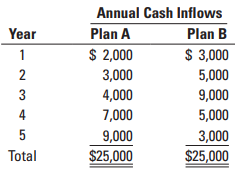### Create an Account

Home / Questions / Exercises in compound interest no income taxes To be sure that you understand how to us...

# Exercises in compound interest no income taxes To be sure that you understand how to use the tables in Appendix A at the end of this book solve the following exercises Ignore income tax

Exercises in compound interest, no income taxes. To be sure that you understand how to use the tables in Appendix A at the end of this book, solve the following exercises. Ignore income tax considerations. The correct answers, rounded to the nearest dollar, appear on page 855.

1. You have just won \$50,000. How much money will you accumulate at the end of 5 years if you invest it at 6% compounded annually? At 12%?

2. Twelve years from now, the unpaid principal of the mortgage on your house will be \$249,600. How much do you need to invest today at 6% interest compounded annually to accumulate the \$249,600 in 12 years?

3. If the unpaid mortgage on your house in 12 years will be \$249,600, how much money do you need to invest at the end of each year at 6% to accumulate exactly this amount at the end of the 12th year?

4. You plan to save \$4,800 of your earnings at the end of each year for the next 8 years. How much money will you accumulate at the end of the 8th year if you invest your savings compounded at 4% per year?

5. You have just turned 65 and an endowment insurance policy has paid you a lump sum of \$400,000. If you invest the sum at 6%, how much money can you withdraw from your account in equal amounts at the end of each year so that at the end of 7 years (age 72), there will be nothing left?

6. You have estimated that for the first 6 years after you retire you will need a cash inflow of \$48,000 at the end of each year. How much money do you need to invest at 4% at your retirement age to obtain this annual cash inflow? At 6%?

7. The following table shows two schedules of prospective operating cash inflows, each of which requires the same net initial investment of \$18,000 now:The required rate of return is 6% compounded annually. All cash inflows occur at the end of each year. In terms of net present value, which plan is more desirable? Show your computations.

Jul 15 2020 View more View LessSubscribe To Get Solution